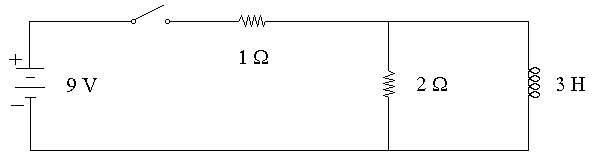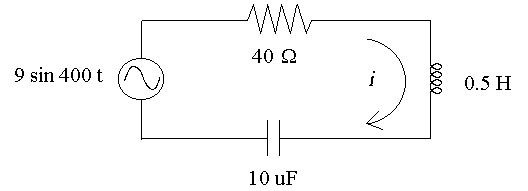First Name: ___________________ Last Name: ____________________ Section: _________

March 21, 1997 Physics 208

Exam 2

Print your name and section clearly on all five pages. (If you do not know your section number, write your TAs name.) Show all work in the space immediately below each problem. Your final answer must be placed in the box provided. Problems will be graded on reasoning and intermediate steps as well as on the final answer. Be sure to include units wherever necessary, and the direction of vectors. Each problem is worth 25 points. In doing the problems, try to be neat. Check your answers to see that they have the correct dimensions (units) and are the right order of magnitude. You are allowed one 8½ x 11" sheet of notes and no other references. The exam lasts exactly 50 minutes.

(Do not write below)

SCORE:

Problem 1: __________

Problem 2: __________

Problem 3: __________

Problem 4: __________

TOTAL: ___________

First Name: ___________________ Last Name: ____________________ Section: _________

1. A solenoid with a length of 30 cm and a diameter of 2 cm has 100 turns of wire distributed uniformly along its length and produces a magnetic field of 20 Gauss in its interior.

a. What is the current in the wire? (5 pts.)

b. What is the magnetic flux in the solenoid? (5 pts.)

c. What is the inductance of the solenoid? (5 pts.)

d. What is the total magnetic energy stored in the solenoid? (5 pts.)

e. What maximum velocity can an electron (me = 9.1 x 10-31 kg) have and be confined within the solenoid? (5 pts.)

First Name: ___________________ Last Name: ____________________ Section: _________

1. A single-turn circular loop of wire with a radius of 10 cm rotates 60 times per second with its axis perpendicular to a magnetic field of 0.5 T.

a. Calculate the peak voltage induced in the loop. (10 pts.)

b. Calculate the rms voltage induced in the loop. (5 pts.)

c. If the loop has a resistance of 2 W , what average power is dissipated in the loop? (10 pts.)

First Name: ___________________ Last Name: ____________________ Section: _________

3. After being closed for a long time, the switch in the circuit below is opened at t = 0.a. What is the current in the 1-W resistor just before the switch opens? (5 pts.)

b. What is the current in the 2-W resistor just before the switch opens? (5 pts.)

c. What is the current in the inductor just after the switch opens? (5 pts.)

d. What is the voltage across the inductor just after the switch opens? (5 pts.)

e. What is the voltage across the inductor at t = 0.5 s? (5 pts.)

First Name: ___________________ Last Name: ____________________ Section: _________

4. In the circuit below, the voltage source is sinusoidal.a. Calculate the peak value of the current i. (15 pts.)

b. Calculate the phase of i relative to the source. (10 pts.)